# Facts, Factors, and a Countdown to 2023

Contents

### Countdown to 2023:

The last 10 seconds of the year, we like to countdown from 10 to the new year. I like a mathematical way of counting down so I try to make an equation with the numbers from 10 to 1 that equals the coming year. This year I could have based my countdown  on last year’s countdown and said
(10-9+8×7×6)(5-4)(3)(2)+1 = 2023,
but this is a blog about factoring so I want a countdown that takes you to the prime factors of 2023 first. Here’s my countdown: (Note: Even though I used 1 as a factor twice in the countdown, I am very much aware that 1 is not a prime factor of any number.)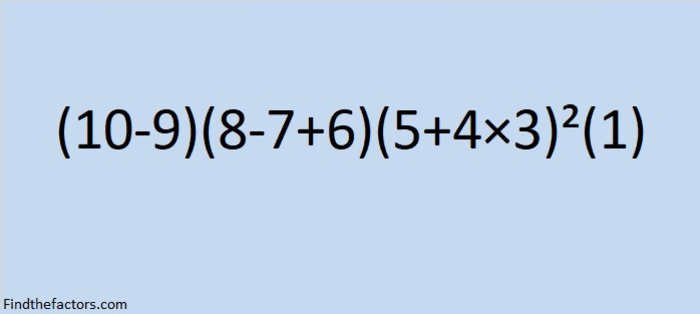make science GIFs like this at MakeaGif
Here’s the final shot from the Countdown: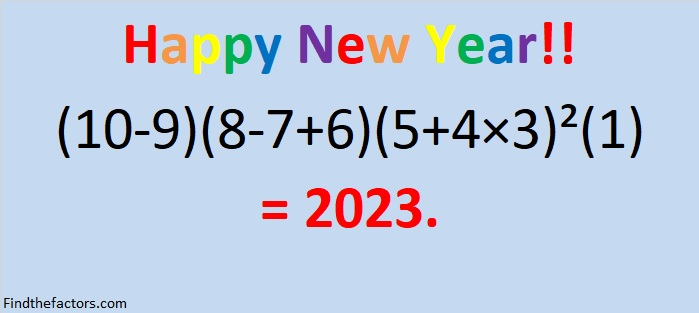### A Puzzle for 2023:

2023 has something in common with 2022. When either number and their reverses are squared, something interesting happens…it’s almost like looking in a mirror!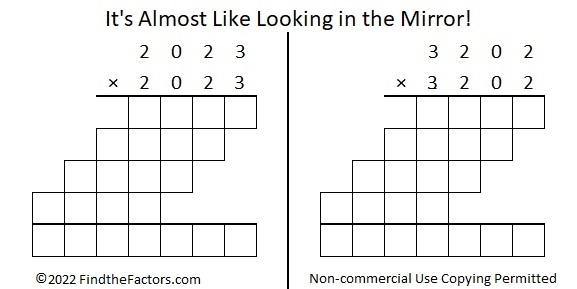Only 50 numbers less than 10000 can make a similar claim to fame:### Factors of 2023:

• 2023 is a composite number.
• Prime factorization: 2023 = 7 × 17 × 17, which can be written 2023 = 7 × 17².
• 2023 has at least one exponent greater than 1 in its prime factorization so √2023 can be simplified. Taking the factor pair from the factor pair table below with the largest square number factor, we get √2023 = (√289)(√7) = 17√7.
• The exponents in the prime factorization are 1 and 2. Adding one to each exponent and multiplying we get (1 + 1)(2 + 1) = 2 × 3 = 6. Therefore 2023 has exactly 6 factors.
• The factors of 2023 are outlined with their factor pair partners in the graphic below.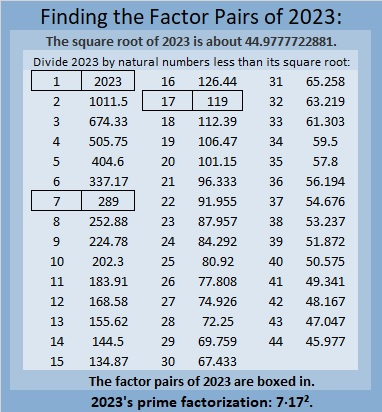### More About the Number 2023:

What do 2023 tiny squares look like?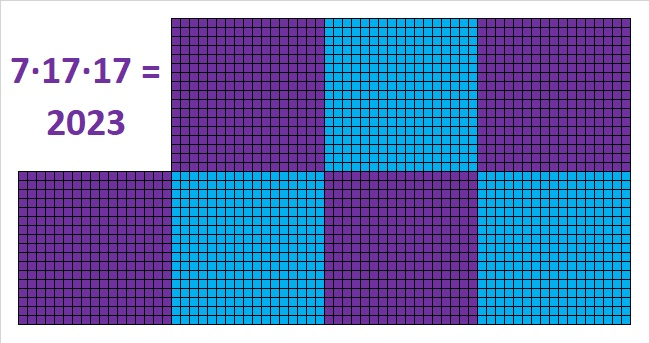2023 is the sum of consecutive numbers in five different ways: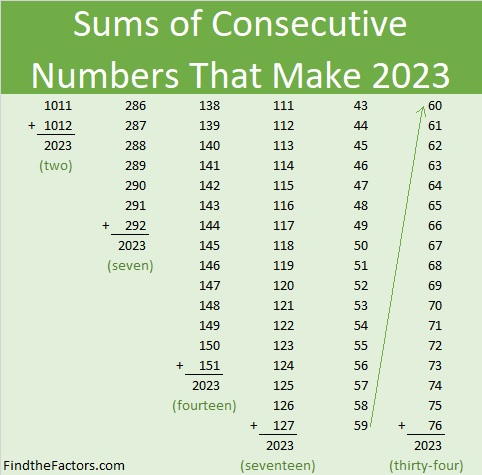And it is the sum of consecutive odd numbers in two ways: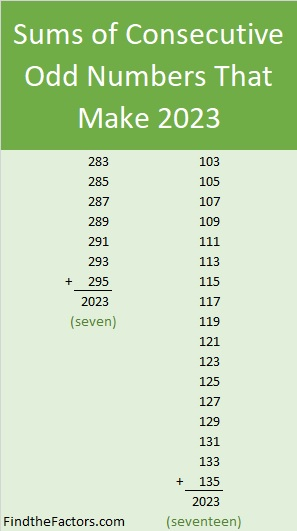2023 is a palindrome in base 16 because
7(16²) + 14(16) + 7(1) = 2023.

This tweet demonstrates that the prime factors of 2023 have a relationship with the digits of 2023.

I saw this tweet on Twitter and immediately recognized something else cool about 2023.

That might seem like a lot of mathematical mumble jumble, but with a little bit of explanation, it can be understood. And even though I made the problem look scarier because I substituted 2+0+2+3 for 7, some older elementary students who already understand powers, factorials, and/or remainders, will get it. I’m confident you can too.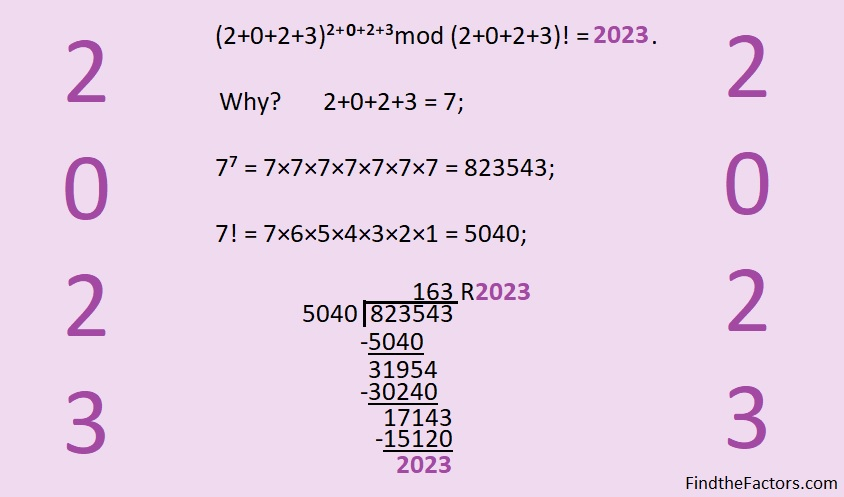You could also give the following list of facts to older elementary students and ask them to use it to find the remainder when they divide 823,543 by 5040.

1×5040 =    5040
2×5040 = 10080
3×5040 = 15120
4×5040 = 20160
5×5040 = 25200
6×5040 = 30240
7×5040 = 35280
8×5040 = 40320
9×5040 = 45360

Because its factors, 17 and 289, are hypotenuses of Pythagorean triples, 2023 is also the hypotenuse of some Pythagorean triples:
952-1785-2023 which is 119(8-15-17) , and
1127-1680-2023 which is 7(161-240-289).

Ureczky József also pointed out in the comments of this post, that 2023 is the short leg in SIX Pythagorean triples, and thus
2023² = 2046265² – 2046264²
2023²= 292327² – 292320²
2023² = 120377² – 120360²
2023² = 17255² – 17136²
2023² = 41785² – 41736²
2023² = 7225² – 6936²

One of those triples is a primitive triple. Can you determine which one?

Ureczky József shared one more amazing fact in the comments that I’m replicating here:

2023=9³+8³+7³+6³+5³+4³+3³+2³-1³

### More Mathematical Tweets About 2023:

These tweets are more or less in the order I saw them, not in order of mathematical difficulty. I will add more as I see them.

v

## 3 thoughts on “Facts, Factors, and a Countdown to 2023”

1.Jozsef Ureczky

2023=9^3+8^3+7^3+6^3+5^3+4^3+3^3+2^3-1^3

•ivasallay

That’s amazing! Köszönön!

2.Ureczky József

2023^2 = 2046265^2 – 2046264^2
2023^2 = 292327^2 – 292320^2
2023^2 = 120377^2 – 120360^2
2023^2 = 17255^2 – 17136^2
2023^2 = 41785^2 – 41736^2
2023^2 = 7225^2 – 6936^2

This site uses Akismet to reduce spam. Learn how your comment data is processed.